Related Articles

# Newton’s Second Law of Motion

• Last Updated : 24 Jun, 2021

Force is a push or a pull that changes or tends to induce a change in the state of the object or it may modify the direction in which the object is moving or transform the shape of an object. The body may be in a state of rest or uniform motion. The application of force induces an acceleration in the object.

The push or pull applied on an object with mass causing it to change its velocity.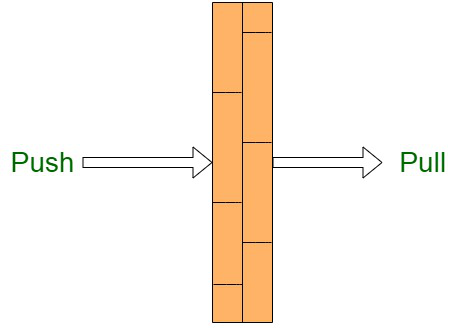Characteristics of force:

• Force is a vector quantity, that is it is associated with both magnitude and direction.
• The S.I unit of measurement of force is Newton, denoted by N.
• Represented by the symbol F.

Effects of Force:

• It can induce a change in the speed of a body.
• It can induce a change in the direction of force and motion of a body.
• It can induce a change in the shape of a body.

Formula for force

The quantity of force(F) can be expressed as a vector product of mass (m) and acceleration (a), since acceleration is a vector quantity.

Mathematically,

F = ma

Where,

m = mass

a = acceleration

The force is measured in Newton (N) or Kg m/s2.

Balanced and Unbalanced Forces

There are two types of forces namely balanced forces and unbalanced forces :

• Balanced Forces

Any object when subjected to equal magnitude forces from different directions, such that the effects of these forces is cancelled out in each other’s presence, is termed as the balanced forces. Since, the objects experience a total cancellation force, the the state of rest or motion of an object remains unmodified. However, it may change the shape or size of an object.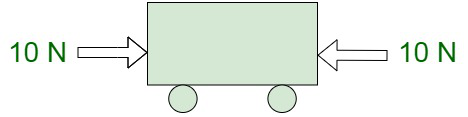• Characteristics of Balanced Forces
• Balanced forces do not cause any change in state of an object.
• Balanced forces are equal in magnitude and opposite in direction.
• Balanced forces may change the shape and size of an object.

Example: When a balloon is pressed from opposite sides, the size and shape of balloon is modified.

• Unbalanced Forces

When forces applied to an object from different directions are of differing magnitude, they can transform the state of rest or motion of an object, that is it may even cause acceleration in an object in the direction of greater force application. They may induce a change in the shape and size of an object.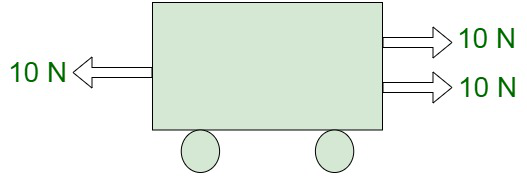• Characteristics of Unbalanced Forces
• Unbalanced forces may change the speed and position of an object.
• Unbalanced forces may change  the shape and size of an object.

Newtons Law of Motion

The branch of physics dealing with the simulated motions of all large-scale and familiar bodies in the universe, for instance, the cars, planets, and humans comes under classical mechanics, stipulated by Newton. In comparison, the motion of minutely small atomic and sub-atomic objects is covered under the branch of quantum mechanics, stipulated by Euler.

The following are the Newton’s Laws of Motion :

• Law of Inertia – Newton’s first law states that, if a body is at rest or moving at a constant speed in a straight line, it will remain at rest or keep moving in a straight line at constant speed unless it is acted upon by an external acting force.
• The second law states that the rate of change of momentum of a body is directly proportional to the force applied for any object of constant mass.
• The third law states that when one object applies a force on a second object, the second object exerts a force back on the first object that is equal in magnitude and opposite in direction.

Second Law of Motion

Isaac Newton’s second law of motion gives the relationship between the force and acceleration of any object in the universe. This postulate states that:

The rate of change of momentum of an object is proportional to the applied unbalanced force, in the direction of force.

The rate of change of momentum of an object is proportional to the applied force.

Mathematically,

Force∝\frac{Change\ in\ Momentum}{Time\ Taken}

Momentum

Momentum of a body is the product of the mass of the body and its associated velocity. Momentum can be considered to be a vector quantity, that is, it has both an associated magnitude as well as direction.  By the second law of motion, the time rate of change of momentum is equal to the force acting on the particle.

By the formula, we have,

Momentum = Mass × Velocity

Let us consider an object of mass, m to be moving along a straight line with an initial velocity, u m/s.

It is uniformly accelerated to velocity, v in time, t by the application of a constant force, F throughout the time, t.

The initial and final momentum of the object will be,

p1 = mu and p2 = mv respectively.

The change in momentum is given by ,

p2 – p1 = m × (v – u)

Rate of change of momentum  =Since, force applied is proportional to rate of change of momentum,

Therefore, we have,………equation (1)

Here,

a =, which is the acceleration which is the rate of change of velocity.

The quantity, k is assumed to be a constant of proportionality.

The unit of force is chosen in such a way that the value of the constant, k becomes equivalent to 1.

For this, one unit of force is defined as the amount that produces an acceleration of 1 ms-2 in an object of 1 kg mass.

That is,

1 unit of force = k × (1 kg) × (1 m s-2).

Thus, the value of k becomes 1.

From equation (1)

F = ma

The unit of force is kg ms-2 or Newton, which has the symbol N (newton) .

The second law of motion gives us a method to measure the force acting on an object as a product of its mass and the associated acceleration.

Derivation for Second law of Motion

We have,

Initial momentum p1 = mu

Final momentum p2 = mv

Therefore,

Change in momentum = p2 – p1 = mv – mu = m(v – u)

Adhering to the second law of motion,

Force F ∝F∝Let us assume, the constant of proportionality,

k = 1

Therefore,

F = ma, which implies that the force is equivalent to the product of mass and acceleration of the body.

For Changing Mass

Let us assume a body to be at an initial point (0) specified at location L0 and at the time instance t0 . Let us assume the body having mass m0 and travelling with a uniform velocity v0 .

On subjecting the body to a force F, the body moves to point 1, situated at the location L1 and reaches this point at time instance t1.

The mass and velocity of the body undergoes transformation as the body travels to v1.

Deriving the values for m1 and v1, we get,

F=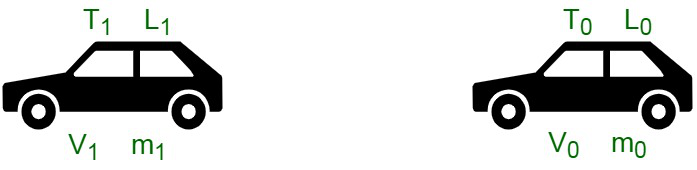For Constant Mass

For a constant mass, Newton’s second law can be equated as follows:Application of Second Law (or second law of motion in everyday life)

The application of the second law of motion can be seen in identifying the amount of force needed to make an object move or to make it stop. Following are a few examples that we have listed to help you understand this point:

1. On the cricket ground, the fielder pulls his hands in the backward direction to catch the fast approaching cricket ball. This reduces the momentum of the ball and induces a delay. When the ball comes in the hand of fielder and comes to a halt, the momentum of ball is reduced to zero. In case, the ball stops suddenly the momentum reaches 0 in an instant time frame. There is a quick rate of change in momentum due to which the player’s hand may get injured. Therefore,  pulling the hand backward a fielder induces delay to the change of momentum to become zero, to prevent injury.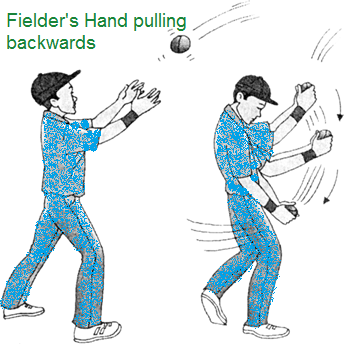2. Kicking a ball – When we kick a ball we exert force in a specific direction, which is the direction in which it will travel. In addition, the stronger the ball is kicked, the stronger the force we put on it and the further away it will travel.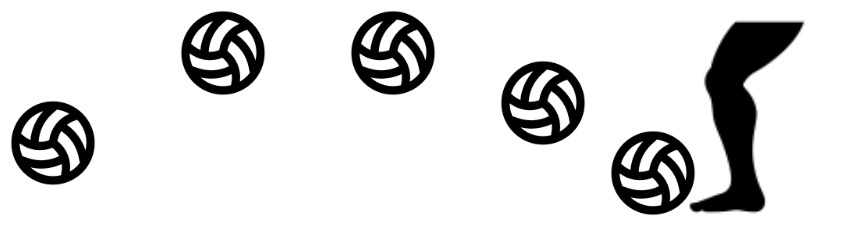3. Pushing a cart – Since, the force is directly proportional to the mass of the object, we can observe that it is easier to push an empty cart in a supermarket than it is to push a loaded one. A heavier mass upon application of the same force, tends to show a smaller acceleration.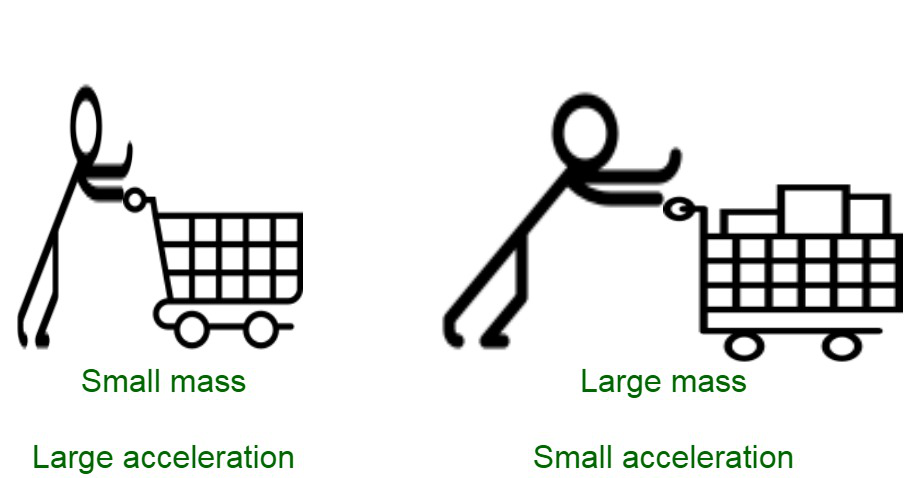4. Two people walking – The heavier person tends to walk slower than the thinner one, upon exerting same force because the acceleration of the person weighing lighter is greater.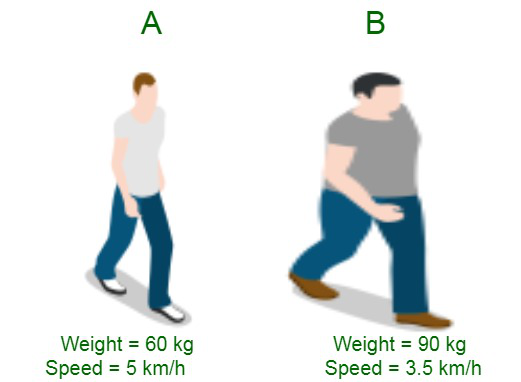5. It is easier to push a car than a truck with the same amount of force as the mass of the car is lesser than the mass of the truck.

6. In golf game, the change in acceleration of the golf ball is directly proportional to the force with which it is hit by the player through a golf stick.

7. The rocket tends to move faster due to the force applied known as thrust and undergoes a change in acceleration and is an example of Newton’s second law of motion.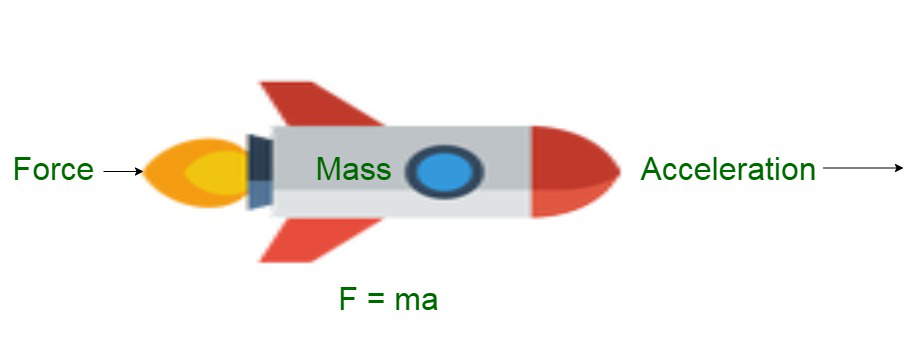### Sample Problems

Example 1. Find out How much net force will be needed to accelerate a 2500 kg truck at 5.50 m/s2?

Solution:

Here we have,

Acceleration (a) = 5.50 m/s2

Mass of the Truck (m)= 2500 kg

Hence,

Force = mass × acceleration

F = 2500 × 5.5

F = 13750 N

Net force will be needed to accelerate a 2500 kg truck at 5.50 m/s2 is 13750 N.

Example 2. What will happen If a net force of 6 N is applied on 0.5 kg-material, Calculate the acceleration of the material?

Solution:

Here we have

Force (F) = 6 N

Mass (m) = 0.5 kg

acceleration (a) = ?

Force = mass × acceleration

F = m × a

a =a =a = 12 m/s2

The acceleration of the material is 12 m/s2

Example 3: What will happen when a net force of 70 N is constantly applied on 1.4 kg body at rest, What time it will take to attain the velocity to 150 m/s?

Solution:

Here we have

Force (F) = 70 N

Mass of the body (m) = 1.4 kg

Initial velocity = 0 m/s (as body is at rest)

Final velocity = 150 m/s

We have to find t = ?

First find the acceleration

Force = mass × acceleration

F = m × a

a =a =a = 50 m/s2

Now use this equation of motion

v = u + at

150 = 0 + (50 × t)

150 = 50 × t

t =t = 3 sec

Example 4. When a train engine of mass 2500 kg is moving with some velocity . If the engine after applying brakes is brought to rest, after application of brakes engine goes about 40m when the average resistance being offered to it is 5000 N. Calculate the velocity of the Train engine?

Solution:

Here we have

Mass of the Train (m) = 2500 kg

Force (F) = – 5000 N   (as negative stopping force is applied)

Distance (s) = 40 m

Final velocity (v) = 0 m/s

We have to find Initial velocity u = ?

Force = mass × acceleration

First find the acceleration

F = m × a

a =a =a = -2 m/s2

Now we will use this equation of motion

v2 = u2 + 2as

0 = u2 + {2 × (-2) × 40}

u = 160 m/s

The initial velocity of the Train engine was 160 m/s

Example 5. If a racing car driver on race track in order to overtake accelerates his racing car first at the rate of 8 m/s2 and then at the rate of 16 m/s2 . Find the ratio of the forces exerted by the engine for the acceleration change?

Solution:

Here we have

a1 = 8 m/s2

a2 = 16 m/s2

We have to find the ratio ofMass of the racing car will be same in both the casesExample 6. If a body of mass 10 kg is moving with a constant velocity of 4 m/s on a frictionless ground. What will be the force required to keep the body moving with the same velocity?

Solution:

Here we have

Mass (m) = 10 kg

acceleration (a) = 0

We have to find F = ?

Force = mass × acceleration

F = 10 × 0

F = 0

Example 7. When a bullet of mass 20 gm is shot from a gun that has an initial velocity of 40 m/s the mass of the gun is 5 kg. What is the initial recoil velocity of the gun?

Solution:

Here we have

mass of bullet (m1) = 20 gm or 0.02 kg

mass of the gun (m2) = 5 kg

Initial velocity = 40 m/s

By the law of conservation of momentum,

0 = 0.02 × 40 + 5 × v

5 × v = -0.8

v =v = -0.16 m/s

Attention reader! Don’t stop learning now. Join the First-Step-to-DSA Course for Class 9 to 12 students , specifically designed to introduce data structures and algorithms to the class 9 to 12 students

My Personal Notes arrow_drop_up